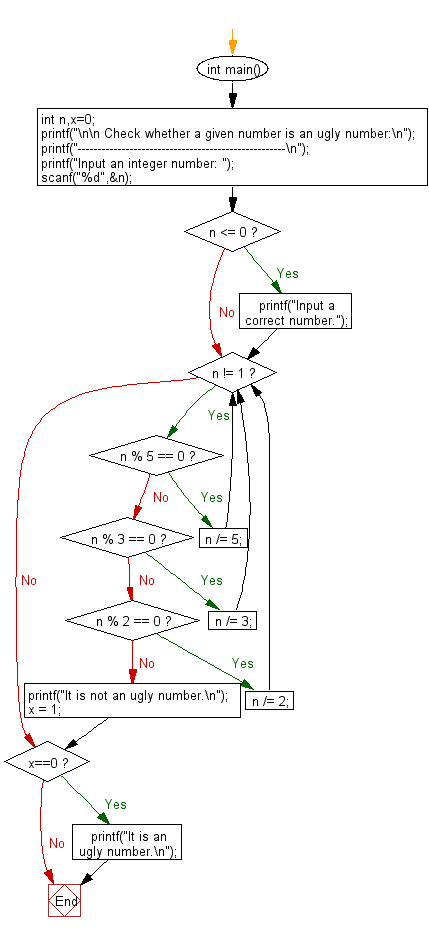﻿ C Program: Check whether a given number is ugly# C Exercises: Check whether a given number is an ugly number

## C Numbers: Exercise-1 with Solution

Write a program in C to check whether a given number is an ugly number or not.

Test Data
Input an integer number: 25

Sample Solution:

C Code:

``````# include <stdio.h>
# include <string.h>

int main()
{
int n,x=0;
printf("\n\n Check whether a given number is an ugly number:\n");
printf("----------------------------------------------------\n");
printf("Input an integer number: ");
scanf("%d",&n);

if (n <= 0) {
printf("Input a correct number.");
}
while (n != 1)
{
if (n % 5 == 0)
{
n /= 5;
}
else if (n % 3 == 0)
{
n /= 3;
}
else if (n % 2 == 0)
{
n /= 2;
}
else
{
printf("It is not an ugly number.\n");
x = 1;
break;
}
}
if (x==0)
{
printf("It is an ugly number.\n");
}
}
```
```

Sample Output:

```Input an integer number: 25
It is an ugly number.
```

Pictorial Presentation:Flowchart:C Programming Code Editor:

What is the difficulty level of this exercise?

Test your Programming skills with w3resource's quiz.

﻿

## C Programming: Tips of the Day

Why do C and C++ compilers allow array lengths in function signatures when they're never enforced?

It is a quirk of the syntax for passing arrays to functions.

Actually it is not possible to pass an array in C. If you write syntax that looks like it should pass the array, what actually happens is that a pointer to the first element of the array is passed instead.

Since the pointer does not include any length information, the contents of your [] in the function formal parameter list are actually ignored.

Ref : https://bit.ly/3fhlvdH